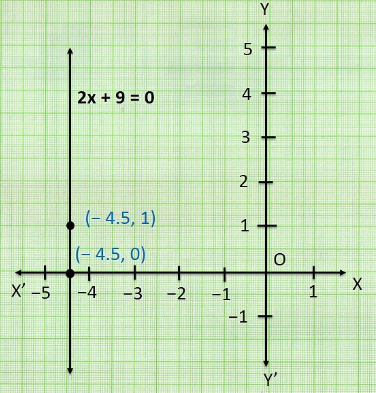Newbie

# Give the geometric representations of 2x+9 = 0 as an equation (ii) in two variables. Q.2(2)

• 0

How i solve the ncert class 9th question of chapter linear equations in two variables of exercise 4.4 question number 2(2). Please give me the simplest and easiest solution of this question  ,also give me the best solution of this question. Give the geometric representations of 2x+9 = 0 as an equation (ii) in two variables.

Share

1. (ii) In two variables,

2x+9 = 0

2x+0y+9 = 0

When y = 0, x = -4.5

When y = 1, x = -4.5• 0